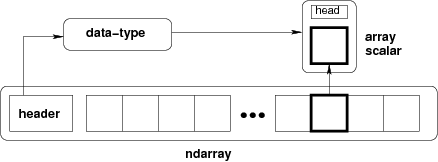NumPy Reference

Next topic

The N-dimensional array (`ndarray`)

Array objects¶

NumPy provides an N-dimensional array type, the ndarray, which describes a collection of “items” of the same type. The items can be indexed using for example N integers.

All ndarrays are homogenous: every item takes up the same size block of memory, and all blocks are interpreted in exactly the same way. How each item in the array is to be interpreted is specified by a separate data-type object, one of which is associated with every array. In addition to basic types (integers, floats, etc.), the data type objects can also represent data structures.

An item extracted from an array, e.g., by indexing, is represented by a Python object whose type is one of the array scalar types built in NumPy. The array scalars allow easy manipulation of also more complicated arrangements of data.Figure Conceptual diagram showing the relationship between the three fundamental objects used to describe the data in an array: 1) the ndarray itself, 2) the data-type object that describes the layout of a single fixed-size element of the array, 3) the array-scalar Python object that is returned when a single element of the array is accessed.